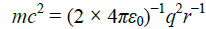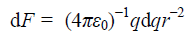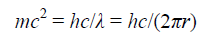All submissions of the EM system will be redirected to Online Manuscript Submission System. Authors are requested to submit articles directly to Online Manuscript Submission System of respective journal.

# Research

, Volume: 7( 3) DOI: 10.37532/2320-6756.2019.7(3).181

## Theoretical Calculation and Proof of Electron and Proton Radius

*Correspondence:
Mei ZH,
College of Chemistry and Molecular Engineering, Qingdao University of Science and Technology, Qingdao, China
Tel: +86-0532-84022681
E-Mail: mzh62@qust.edu.cn

Received: May 11, 2019; Accepted: June 3, 2019; Published: June 10, 2019

Citation: SY Mei, ZH Mei. Theoretical Calculation and Proof of Electron and Proton Radius. J Phys Astron. 2019;7(2):181.

### Abstract

The radius of matter particles plays an important role in nuclear physics and astrophysics. According to Kaluza-Klein and B. Feng’s theory, the exact radii of electron and proton are obtained. Their correctness has been proofed by different ways. It showed us a fact and curious to common that, the size of the more massive proton is much smaller than that of the light electron. The internal motion of charge is also revealed. As theoretical needs, the coefficients of fractal dimensional space are proposed. All these information can’t be obtained from the standard model of elementary particles or those researches accordingly

## Introduction

The electron and proton radius are key problems in elementary particle physics, nuclear physics, and astrophysics. There are two main types of valuable attempts in this respect from different perspectives. All these attempts have some rationality, but not comprehensive. In this work, their faults are analyzed and a convincing theoretical calculation scheme is proposed.

## Methods

Previous attempts

Repulsive energy and classical Lorentz radius of the electron: Classical electron radius of 1.4089698 × 10−15 m was known as the Lorentz radius. It supposed that the total energy (mec2) of a quiet electron comes from the repulsive potential energy of the uniformly distributed charge, which spreading homogeneously in the spherical electron. By integral operation of the homogeneous charge, the relationship and results were obtained as(1)= 1.4089698 × 10−15 m(1.1)

Generally, the electron was considered a point particle with a point charge and no spatial extent, its result has been described as ill-conceived to many people. On the other hand, the charge (q) of the electron is as a whole inseparable; in common sense, we also can’t sure whether it can be treated as uniformly distributed in the sphere even it has a spatial volume; it is difficult to understand the repulsive force comes from itself. The third, even if the repulsive potential energy exists, we still can’t confirm that it is right equal to mec2.

Therefore, it is hard to believe whether the deduced classical electron radius is right or not. Nevertheless, its result looks realistically.

By the way, the classical electron radius as commonly known and present on “Wikipedia” website is 2.8179403227(19) × 10−15 m; whereat, a factor 1/2 is missing; a physical textbook by Fudan University (on page 114) has detailed the case .

Compton radius of the electron: Another attempt was concerning the Compton radius. The expression was as follows for an electron at rest,(2)Where ‘r’ is a variable. It will decrease with the increase of rest mass of a particle.

The Compton’s attempt is obtained according to the Kaluza-Klein’s theory, where a rest elementary particle is regarded as an actually confined motion of light.

Because Klein’s quantum interpretation is not accepted in physics circles and the radius of the result seems much larger than imagines; it is also not been recognized.

Attractive energy and force with inward magnetism: The macroscopic electromagnetic interaction is in four-dimensional space, while the internal motion of elementary particle the five-dimensional. Their energy relation is studied by B. Feng and described in detail in reference . It takes the form of,(3)(3.1)

Where the magnetic field attracts the moving charge inward; the coefficient k1 is explained the next.

Confirmation of the Radius of Matter Particles

Calculating proof of electron radius: We insist on that Kaluza-Klein’s idea is right. So, the second attempt for electron radius of Compton’s has certain rationality; merely it has a missing part. It hasn’t realized that the light confined in a circle is the light propagating motion in five-dimensional space, that possessing energy in five-dimensional; however, mec2 is the energy in three-dimensional space. So, the variable modulus effects of dimensions must be considered. The coefficient of variable modulus is the ratio of the n-dimensional spherical area (Sn) to (n−1) dimensional volume (Vn−1) for dimension n to (n−1) variable modulus. They have formed as,Where the coefficient of radius variable modulus is R/r =Additionally, the inner motion of the electron is not in exactly five-dimensional space, but fractal dimensioned by coefficient k, and k1=0.9781465420 for the fifth dimension which we have ever used in calculating the mass and charge of the electron [3,4], k2=0.9854075625 for the fourth dimension. Therefore, the fractal dimension effects must also be considered.

Considering above variable modulus and fractal dimension effects, (2) should be rewritten as,(4)The result is coincident with that in (1), they are exactly equal. Amazingly that, from the completely different ways, we have come to the same result of electron radius by inserting coefficients k1 and k2; moreover, they are approximately equal to 1. It convinced us the electron radius is to be exact 1.4089698 × 10−15 m. Of course at present view, it is inaccurate and arbitrary to assume the radius of an electron to be equal to neutron, which we ever used before in .

Frankly speaking, the coefficients k1 and k2 are acted as fitting factors in a simple view. But we find that they occurred in many cases; they are indeed the theoretical needs in our theory. So, they should be the new constants in physics. They are more like the fractal dimension coefficients; in my previous paper [2,4], k1 was once called as a spatial bending factor.

Calculation of proton radius: The proton radius (rp) calculating is easy. According to (4), it has the relationship and results as,This result updates the previous value of 1.37036 × 10−18 m .

Neutron radius: Neutron is a composite particle composed of an electron and a proton. Its actual value of radius is the measure of 2.51620 × 10−15 m as we used in . This shows that inserting a proton into an electron can increase the radius of an electron to about twice its size.

## Results and Discussion

Wave nature of elementary particles

Above different attempts have come to the same electron radius. In (1) the classical electromagnetic field interaction laws are applied. The repulsive force of static electricity in (1.1) has been balanced by the attraction force of the current Lorentz force in the magnetic field of Biot-Savant law in (3.1); they are exactly equal. This success shows the conversion mechanism between photon and elementary particle and annotates Klein’s quantum interpretation of elementary particle. In short, the elementary particle is thoroughly a wave.

By the way, though the charge is a whole inner motion and indivisible, however, their charge effects are mathematically differentiable and integrable even in space size of the order of magnitude of 10−19 m; this can’t be misunderstood.

Non-rigid character and formation mechanism

Although the exact electron and proton radius are given above, it not means its rigidity. Because it is an indeed wave packet, the radius merely corresponds to its statistical meaning like that of the electron cloud in the hydrogen atom. In the other hand, we have seen that the homogeneous charge is not static, but with no rest mass moving in the speed of light in the sphere with the equivalent radius; the mass is its overall energy description. We are glad to see a wonderful mechanism from (1), (2) and (3), in which a photon curled up in a circle becomes a charged elementary particle. Or to say that curled up electromagnetic wave becomes an elementary particle; the energy of stopped light converted into the interaction of electromagnetic field, which appearing electrically to the outside, magnetically the inside; the electric and magnetic characters are separated but interaction. It looks like that the homogeneous charge (or the light charge) has enough high permeability, which shields off the inside magnetic field perfectly. It must be mentioned that the charge of electron or proton is not distributed on the surface but in volume. They are actually volume charged.

From the perspective of general relativity

From the perspective of general relativity, the speed of light is constant. However, the above described inner motion mechanism of elementary particles are the curled up propagating light. That is to say, space is bent in the words of general relativity; it can be said as the simplest small Black Hole in a sense. To common people, it is curiously that as we have seen, is not the mass but the strong electromagnetic field that caused the space-bending; however, it fits our theory.

As the theoretical needs mentioned above, the high-dimensions must be fractal by coefficient k, for the fifth dimension the coefficient k equals to 0.9781465420, the fourth dimension the 0.9854075625, for the three-dimension it equals to 1. Why is that? It leaves a question in physics.

Updating former theoretical data of strong interaction force

According to our new neutron model and the new nuclear model , the strong interaction force in nuclear has been calculated in the literature . Because of my carelessness, there was a mistake in it. As the structure of nuclear is steric, according to crystallographic principles, the neutrons in the nucleus should form a tetrahedral compact accumulation, the proton ought to interact with 4 neutrons rather than 3. So, the correct result of nuclear binding energy is,The result is satisfactory. It is very close to the actual measurement value of 2.23 MeV.

Updating former theoretical data of the density of the singularity

As we have obtained the new proton radius, the former calculated singularity density of the Big Bang should be updated by the value of 1.76753 × 1024 g/cm3, which is 5.69541 times than the previous .

## Conclusion

The electron and proton equivalent radius is proofed to be 1.4089698 × 10−15 m and 7.6734895 × 10−19 m, respectively. The neutron radius remains as measured of 2.51620 × 10−15 m. For a simplicity and clarity expression, they are listed as follows:### References

1. Textbook Compiling Group of Fudan University, Physics. People's Education Press (1st Edition, Chinese), Beijing. 1979;2:114.
2. Mei ZH. Fine-structure constant as a pure geometric number among physical background LJRS: Natural and formal. 2019;19:59-62.
3. Mei ZH. B. Feng’s theory: The prediction of the mass spectrum of elementary particles and the confidence of at least 4-d space-time (Part 1). J Phys Astron. 2017;5:126-32.
4. Mei ZH. Only one absolute value of electric charge in matter world. ISROJ. 2019;4:84-5.
5. Mei ZH, Shi JB. Feng’s theory (Part 3): Neutron model and nuclei force mechanism. J Phys Astron. 2018;6:143-49.
6. Mei ZH. After neutron was compressed: The big bang. J Phys Astron. 2018;6:147-49.
Welcome Message

Select your language of interest to view the total content in your interested language

###### Citations : 272

Journal of Physics & Astronomy received 272 citations as per Google Scholar report

#### Indexed In

• Open J Gate
• China National Knowledge Infrastructure (CNKI)
• Cosmos IF
• Directory of Research Journal Indexing (DRJI)
• MIAR
• Secret Search Engine Labs
• Euro Pub
• clarivate-web-of-science-logo-vector
• ICMJE

View More

### 9th International Conference on Physics

Edinburgh, Scotland

### 6th International Conference on Astronomy, Astrophysics and Space Science

Budapest, Hungary

Berlin, Germany

### 9th International Conference on Physics

Edinburgh, Scotland

### 6th International Conference on Astronomy, Astrophysics and Space Science

Budapest, Hungary

### 10th International Conference on Theoretical and Applied Physics